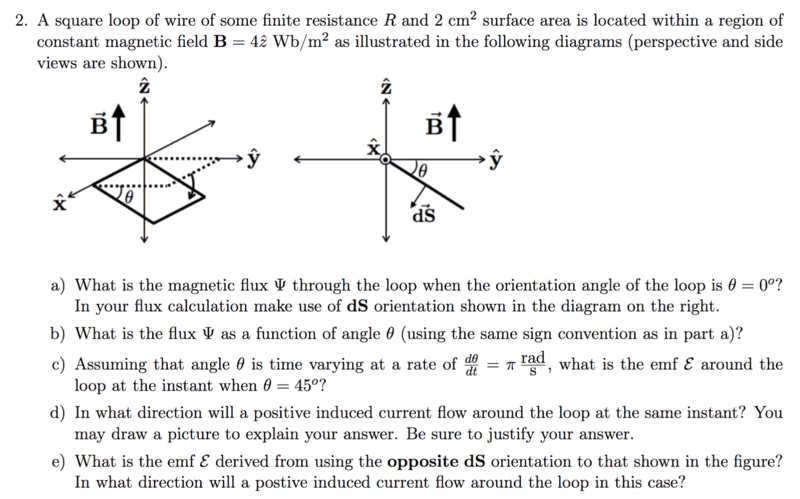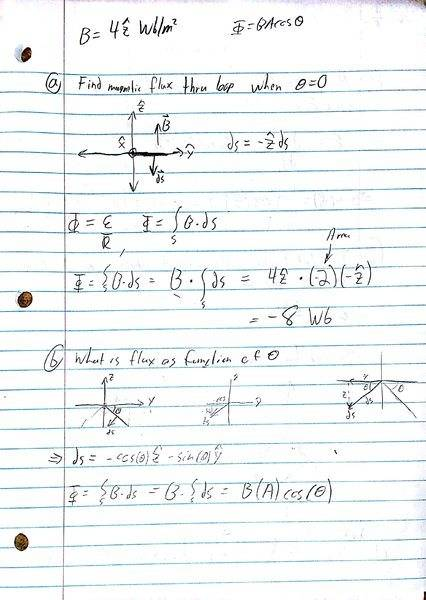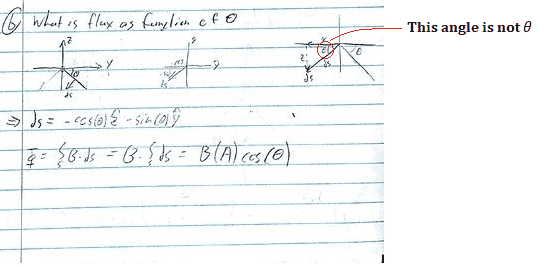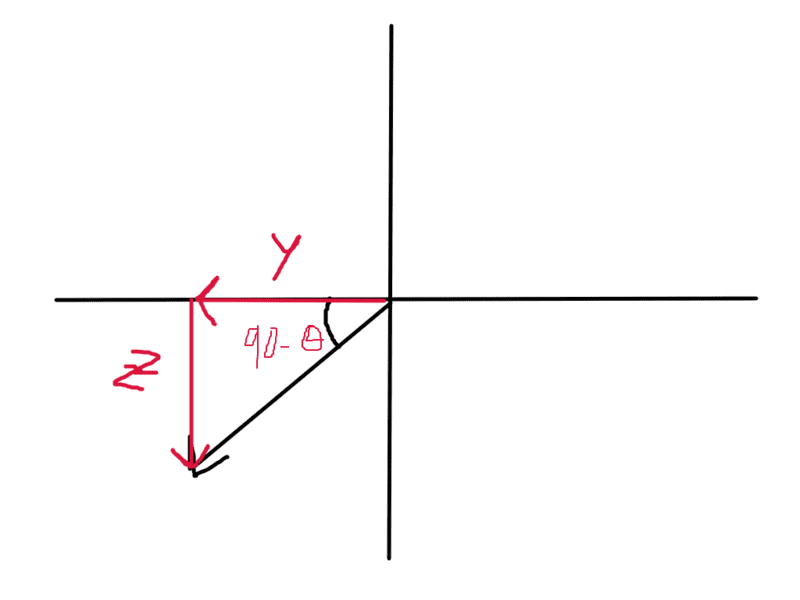# Flux integrals & ds vector

## Homework StatementPart b and e.

## Homework Equations

Flux = surface integral of B (dot) ds

## The Attempt at a Solution

I just want to make sure that I have a good understanding of ds. ds is just the direction of the vector that is normal to our area that we are finding our flux throuhg correct? so that is why in part A we just take B*A in the -z(hat) direction because that is the direction of our ds vector. But for part b we have to find our flux in terms of the angle theta and I am stuck with how to find ds. what I have written down at the bottom there for ds is what the what it should be supposedly, but I can't see where it came from and I am struggling to derive it. How do I get ds here in terms of theta?TSny
Homework Helper
Gold MemberAfter correcting for the mistake shown above, can you find the y and z components of ##\vec{ds}##?

View attachment 212541
After correcting for the mistake shown above, can you find the y and z components of ##\vec{ds}##?
I had a feeling that angle wasn't theta. I am not too sure how to fix that drawing there to solve for ds. if that angle is not theta, what is it?

Edit* is it 90˚ - theta?

TSny
Homework Helper
Gold Member
is it 90˚ - theta?
Yes.

Yes.
so to find the 2 components of ds do we just use cos and sin?==> ds_y = -ds*cos(90-theta)
==> ds_z = -ds*sin(90-theta)

===> ds = -ds[cos(90-theta)+sin(90-theta)]

What about the 90˚ part? how do we get rid of that?

ohhhhhhhh ok got it. That was tricky. Thanks!

I also was a bit confused by part D and E. So we have a initial flux from the surrounding Bfield going through the surface. That flux will induce a current in the wire. Now the question is which direction is it. I thought that the current should go in the direction that will produce an b field that goes against the initial B field. So by that the induced current would be clockwise. But I don't think that is correct and I am not sure why.

TSny
Homework Helper
Gold Member
So we have a initial flux from the surrounding Bfield going through the surface. That flux will induce a current in the wire.
It's not the flux that induces a current, it's the changing flux that induces a current.

Now the question is which direction is it. I thought that the current should go in the direction that will produce an b field that goes against the initial B field.
The B field produced by the induced current does not necessarily point "against" the initial field.

The induced field creates a flux through the loop that opposes the change in initial flux. Another way to say it is that the induced flux is in a direction such as to try to keep the flux constant. Thus if the initial flux increases, the induced flux will be in the opposite direction of the initial flux. But if the initial flux decreases, the induced flux will be in the same direction as the initial flux.